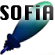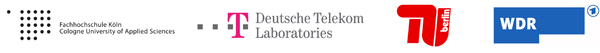Welcome to SOFiA
Who is behind SOFiA
Feature overview
System overview
Function reference
mergeArrayData
F/D/T
gauss
lebedev
S/W/G
S/T/C
W/G/C
S/F/E
M/F
R/F/I
P/D/C
I/T/C
makeMTX
makeIR
visual3D
Coordinate System
Application Examples
Example 1
Example 2
Example 3
Example 4
Example 5
Example 6
Example 7
Example 8
Array Datasets
VariSphear system
Groups and Mailinglists
Contact and Support
How to Reference

# SOFiA Spatial Transform Core

SOFiA S/T/C is a Spatial Fourier Transform (SFT) core optimized for sound field analysis.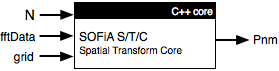## ARGUMENTS

Input

Name Type Purpose Default
N int Maximum transform order -
fftData complex float mtx

Frequency domain data e.g. from F/D/T

-
grid float mtx AZ1 EL1 W1 AZ2 EL2 W2 ... AZM ELM WM for M Positions. Refer to Coordinate System -

Output

Name Type Purpose
Pnm complex float mtx Spatial Fourier coefficients

## Definitions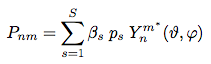Where Y[n, m] describes the Spherical Harmonics of order n and modus m. Beta are the grid weights and p the complex sound pressures

## FILE(S):

 File Type OS/Matlab sofia_stc.m Help header All OS sofia_stc.mexmaci64 MEX core OS-X Intel sofia_stc.mexw32 MEX core Windows, Matlab32 sofia_stc.mexw64 MEX core Windows, Matlab64 sofia_stc.cpp C/C++ source All OS

## Warnings

If the S/F/T core crashes it might be necessary to typecast fftData to double before calling the extermal:
fftData = cast(fftData, 'double');

### Performance

The SOFiA transform corres S/T/C and I/T/C offer a high computation speed and a stable and precise transform at the same time.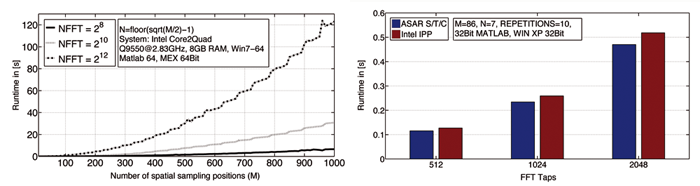Two performance examples of the S/T/C core. The left plot shows the runtime for transform on different FFT blocksizes for a rising number of sensors M at a maximum order N = floor(sqrt(M/2)-1). The FFT blocksize has a linear influence on the runtime. The right plot shows a comparison to the high performance transform algorithm of the Intel Performance Primitives (IPP). The IPP transform is made for computer graphics rendering and not optimized for sound field analysis. The IPP transform can only run up to N=15 and delivers real-valued coefficients that have to be converted to complex ones. The S/T/C core is not order-limited and runs even faster than the IPP transform for sound field analysis.

 ```/* Pnm = sofia_stc(N, fftData, grid)``` `------------------------------------------------------------------------------------` ``` Pnm``` ```Spatial Fourier Coefficients Columns: nm coeff Rows: FFT bins``` `------------------------------------------------------------------------------------` `N` `Maximum transform order` ``` fftData``` ```Frequency domain soundfield data, e.g. from sofia_fdt() Columns: number of spatial sampling positions Rows: FFT bins (complex sound pressure data) ! WARNING cast fftdata to double if core crashes: fftData = cast(fftData, 'double');``` `grid` ```Sample grid (Positions and Weights) Columns: s=1...S spatial sampling positions Rows: [AZ_s EL_s GridWeights_s] AZ in [0...2pi] and EL [0...pi] in RAD``` `*/`